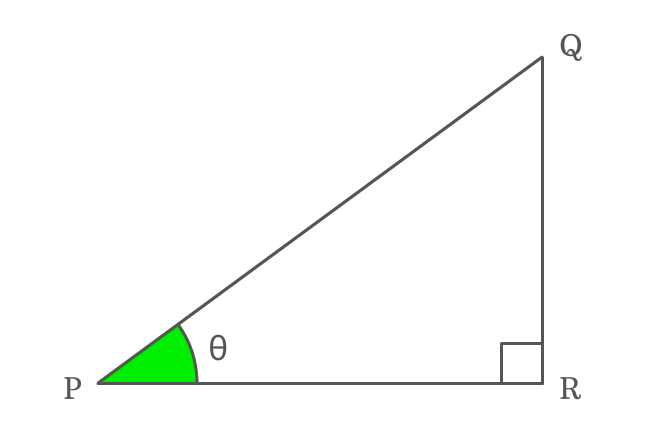# Reciprocal identity of Cos function

## Formula

$\sec{\theta} \,=\, \dfrac{1}{\cos{\theta}}$

### Proof

Cosine is a ratio of lengths of adjacent side to hypotenuse and the secant is a ratio of lengths of hypotenuse to adjacent side. The cosine and secant functions are mutually reciprocals. Therefore, the reciprocal of cos of angle equals to secant of angle.

$\Delta QPR$ is a right triangle and its angle is theta ($\theta$).#### Write cosine in its ratio form

First of all, write cos of angle theta ($\cos{\theta}$) in its ratio form.

$\cos{\theta} \,=\, \dfrac{PR}{PQ}$

#### Write secant in its ratio form

In the same way, write the secant of angle theta ($\sec{\theta}$) in its ratio form.

$\sec{\theta} \,=\, \dfrac{PQ}{PR}$

#### Relation between cosine and secant

Now, write the value of secant function in ratio form into its reciprocal form mathematically. It helps us to identity the relation between cosine and secant functions.

$\implies \sec{\theta} \,=\, \dfrac{1}{\dfrac{PR}{PQ}}$

$\,\,\, \therefore \,\,\,\,\,\,$ $\sec{\theta} \,=\, \dfrac{1}{\cos{\theta}}$

Therefore, it has proved that the reciprocal of cos function is equal to secant function. It is used as a trigonometric formula in mathematics.

###### Note

Any symbol can be used to represent angle of right triangle but the reciprocal identity of cos function should be written in terms of corresponding angle.

For example, if angle of right triangle is denoted by $x$, then

$\sec{x} \,=\, \dfrac{1}{\cos{x}}$

Similarly, if angle of right triangle is represented by $A$, then

$\sec{A} \,=\, \dfrac{1}{\cos{A}}$

The reciprocal identity of cos function is written in this form but the only changing factor is angle of the right triangle.

Latest Math Topics
Jun 26, 2023
Jun 23, 2023

Latest Math Problems
Jul 01, 2023
Jun 25, 2023
###### Math Questions

The math problems with solutions to learn how to solve a problem.

Learn solutions

Practice now

###### Math Videos

The math videos tutorials with visual graphics to learn every concept.

Watch now

###### Subscribe us

Get the latest math updates from the Math Doubts by subscribing us.